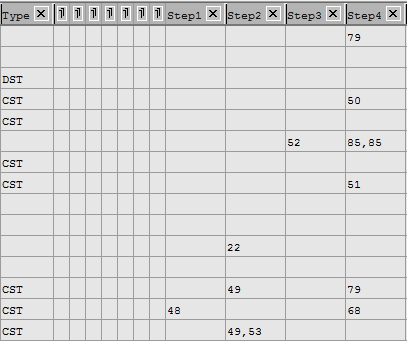# New to QlikView

Discussion board where members can get started with QlikView.

Contributor III

## Counting number of rows with multiple conditions

Dear Qlikview Experts,

My data model was pasted in below:How can I count the correct number of rows if my 'Type' field is = DST or CST and my 'Step1 - Step4' fields doesn't contain any numbers?

So my desired outcome would be 3 rows?

Thank you for your time in advance!Best Regards

1 Solution

Accepted SolutionsMVP

## Re: Counting number of rows with multiple conditions

In the back end

=Count({<Type = {'CST', 'DST'}>} If(Len(Step1) or Len(Step2) or Len(Step3) or Len(Step4), Null(), Type))

But a better approach is to create a flag field in the load script

If(Len(Step1) or Len(Step2) or Len(Step3) or Len(Step4), 0, 1) as FlagEmpty,

...

And then use expression

=Count({<Type = {'CST', 'DST'}, FlagEmpty = {0}>} Type)

Logic will get you from a to b. Imagination will take you everywhere. - A Einstein
7 RepliesMVP

## Re: Counting number of rows with multiple conditions

At the back-end or UI?

Contributor III

## Re: Counting number of rows with multiple conditions

Dear Tresesco B,

It was at UI.

My current expression looks like this (Where ProductNumber, Date & Time are my other fields):

count(aggr(if(Rangesum(if(Len(Step1)=0,1),if(Len(Step2)=0,1),if(Len(Step3)=0,1),if(Len(Step4)=0,1),if(Type='DST',1))=5,1),ProductNumber,Date,Time))

However, I was facing problems in adding 'or' into my 'Type' field. Is there any suggestions?Best RegardsMVP

## Re: Counting number of rows with multiple conditions

In the back end

=Count({<Type = {'CST', 'DST'}>} If(Len(Step1) or Len(Step2) or Len(Step3) or Len(Step4), Null(), Type))

But a better approach is to create a flag field in the load script

If(Len(Step1) or Len(Step2) or Len(Step3) or Len(Step4), 0, 1) as FlagEmpty,

...

And then use expression

=Count({<Type = {'CST', 'DST'}, FlagEmpty = {0}>} Type)

Logic will get you from a to b. Imagination will take you everywhere. - A EinsteinMVP

## Re: Counting number of rows with multiple conditions

If ProductNumber is an identifying* field for the table, then a pure set analysis expression could be

=Count({<Type = {'CST', 'DST'}, ProductNumber = {"\$(=(Len(Step1) or Len(Step2) or Len(Step3) or Len(Step4)) = 0)"} Step)

* a field whose values uniquely identify a row in the table, like a primary key or unique index in SQL.

Logic will get you from a to b. Imagination will take you everywhere. - A Einstein
Honored Contributor II

## Re: Counting number of rows with multiple conditions

You can create a straight chart at UI and set Type as Dimension and below as expression:

Or in a text box as you required,

sum(if( (Type ='DST' or Type=CST') and len(trim(Step4))=0 and len(trim(Step3))=0,1,0))

BR,

KC

Best Regards,
KC
Contributor III

## Re: Counting number of rows with multiple conditions

Dear Jonathan,

Thank you so much for the help! Your expression is better than my original one! Thank you!

Best Regards

QianNing

New Contributor II

## Re: Counting number of rows with multiple conditions

I am trying to count with two conditions and getting results based only on the first condition.

=Count( DISTINCT {<A-={"DC"}>} {<B-={"KC"}>} A&'|'&B)

Can you help with this?

I want OR operator between the two conditions.